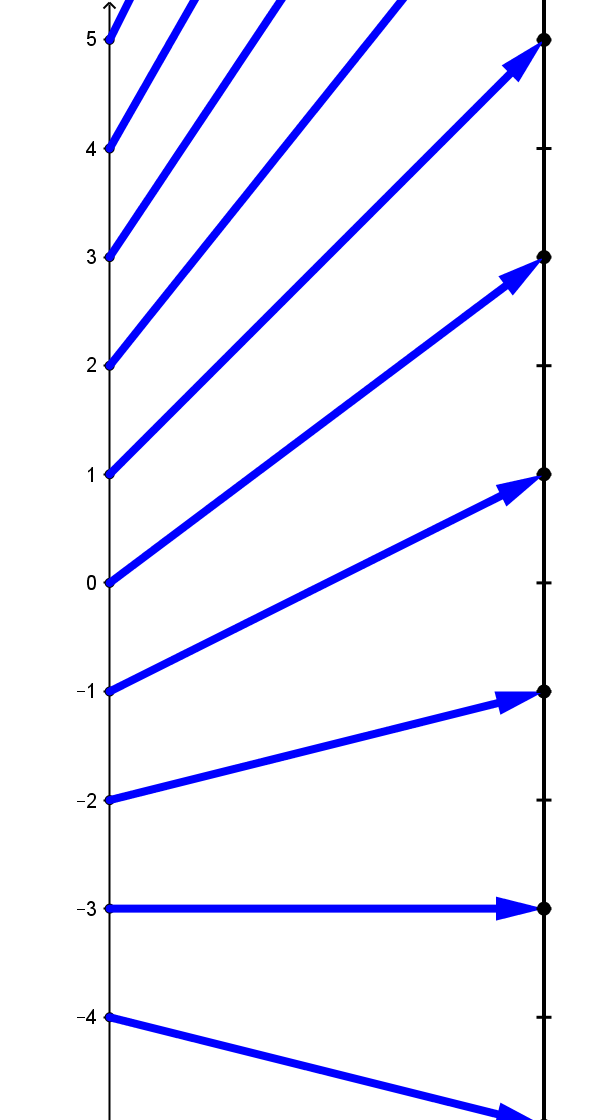### SectionVFIntroduction to Mapping Diagrams: Visualizing Functions

Visualizing Functions: An Overview *

Introduction: The more one studies mathematics, the more one encounters the concept of a function. By talking about functions we come to understand how they encode information. To bring a deeper understanding to functions we give them visual expression. Seeing the information of a function presented in a variety of forms helps us recognize more concretely some of its fundamental features.

These resource materials have been developed to help students understand functions more sensibly  and deeply using four common ways to present the encoded information of a function. Students may have encountered some of these methods previously, but in this resource you will  see how each of these methods give us different ways to view the same underlying relation.

The four ways that connect an understanding of functions are:

• Tables of data for "variable" quantities.
• Algebraic equations that determine a method for determining the value of (controlled or dependent) variable quantities uniquely from the value of other (controlling or independent) variable quantities.
• The visualization of data for  variable quantities using the Cartesian coordinate system.
• The visualization of data for variable quantities using mapping diagrams.Mapping Diagram TMD1

Other Names: Mapping diagrams are also described as function diagrams, dynagraphs, parallel coordinate graphs, or cographs.

See the Bibliography for other historical and current relevant resources.

This resource will provide you with examples, explanations, exercises and problems that will help students use the power of the mapping diagram  along with the three other tools to understand functions.

##### VF.MDIMapping Diagrams are Important.

The key idea in visualizing real valued functions of one variable with mapping diagrams is to have two parallel number lines (or axes), one line  representing the source (input, controlling or independent) variable values and the other line representing the target (output, controlled or dependent) variable values. The function is visualized by arrows that relate points (numbers) on these parallel axes.

#### Mapping Diagram Definition

Using some dynamic technology with GeoGebra we can see connections between the graph and mapping diagram visualizations.

#### Connecting Mapping Diagrams and Graphs

Example TMD.0 The First Example.
This example presents a single function using an algebraic formula, a table of data,  a graph and a mapping diagram.
You can download and try the worksheet for this section now: Worksheet.VF1.pdf.